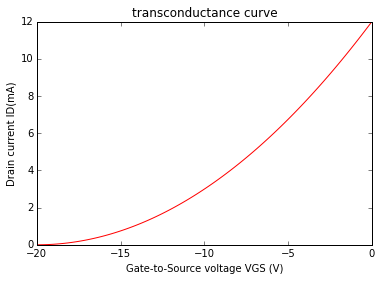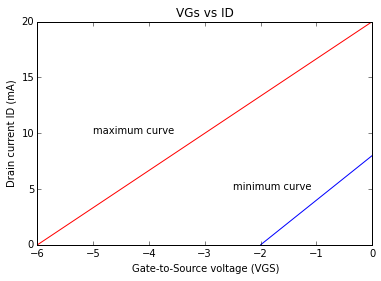# Chapter 16 , Field-Effect Transistor¶

## Example 16.1 , Page Number 350¶

In :
import math

#Variables

IDSS = 15.0                            #Drain-Source current (in milli-Ampere)
VGSoff = -5.0                          #Gate-Source voltage (in volts)

#Calculation

#When VGS = 0 volts
VGS1 = 0                               #VGS (in volts)
ID1 = IDSS * (1 - (VGS1 /VGSoff)**2)   #Drain current (in milli-Ampere)

#When VGS = -1 volt
VGS2 = -1                              #VGS (in volts)
ID2 = IDSS * (1 - VGS2 /VGSoff)**2     #Drain current (in milli-Ampere)

#When VGS = -4 volt
VGS3 = -4                              #VGS (in volts)
ID3 = IDSS * (1 - VGS3 /VGSoff)**2     #Drain current (in milli-Ampere)

#Result

print "Drain current when VGS = 0 V is ",ID1," mA.\nDrain Current when VGS = -1 V is ",ID2," mA.\nDrain Current when VGS = -4 V is ",ID3," mA."

Drain current when VGS = 0 V is  15.0  mA.
Drain Current when VGS = -1 V is  9.6  mA.
Drain Current when VGS = -4 V is  0.6  mA.


## Example 16.2 , Page Number 350¶

In :
import numpy
%matplotlib inline
from matplotlib.pyplot import plot,title,xlabel,ylabel

#Variables

VGSoff = -20.0                          #Gate-Source voltage (in volts)
IDSS = 12.0                             #Drain-Source current (in milli-Ampere)

#Calculation

#When VGS = -5 V
VGS1 = -5                               #VGS (in volts)
ID1 = IDSS * (1 - VGS1/VGSoff)**2       #Drain current (in milli-Ampere)

#When VGS = -10 V
VGS2 = -10                              #VGS (in volts)
ID2 = IDSS * (1 - VGS2/VGSoff)**2       #Drain current (in milli-Ampere)

#When VGS = -15 V
VGS3 = -15                              #VGS (in volts)
ID3 = IDSS * (1 - VGS3/VGSoff)**2       #Drain current (in milli-Ampere)

#Result

print "Drain current when VGS = 0 V is ",ID1," mA.\nDrain Current when VGS = -1 V is ",ID2," mA.\nDrain Current when VGS = -4 V is ",ID3," mA."

#Graph

x = numpy.linspace(-20,0,100)
y = x
plot(x,12*(1+y/20)**2,'r')
title("transconductance curve")
xlabel("Gate-to-Source voltage VGS (V)")
ylabel("Drain current ID(mA)")

Drain current when VGS = 0 V is  6.75  mA.
Drain Current when VGS = -1 V is  3.0  mA.
Drain Current when VGS = -4 V is  0.75  mA.

Out:
<matplotlib.text.Text at 0x6113d50>## Example 16.3 , Page Number 351¶

In :
import math
import numpy
%matplotlib inline
from matplotlib.pyplot import plot,title,xlabel,ylabel,annotate

#Variables

VGSoffmin = -2.0                          #Gate-Source voltage (in volts)
VGSoffmax = -6.0                          #Gate-Source voltage (in volts)
IDSSmin = 8.0                             #Drain-Source current (in milli-Ampere)
IDSSmax = 20                              #Drain-Source current (in milli-Ampere)

#Calculation

#ID = IDSS * (1 - VGS/VGSoff)**2           #Drain current (in milli-Ampere)

#Plotting different values of VGS in the graph

#Result
print "The maximum curve and minimum curves are plotted as shown in the following plots."

#Graph

x1 = numpy.linspace(-2,0,2)
y1 = x1
plot(x1,8 * (1 + y1/2)**2)
x2 = numpy.linspace(-6,0,2)
y2 = x2
plot(x2,20 * (1 + y2/6)**2,'r')
title("VGs vs ID")
xlabel("Gate-to-Source voltage (VGS)")
ylabel("Drain current ID (mA)")
annotate("maximum curve",xy=(-5,10))
annotate("minimum curve",xy=(-2.5,5))

The maximum curve and minimum curves are plotted as shown in the following plots.

Out:
<matplotlib.text.Annotation at 0x6249bd0>## Example 16.4 , Page Number 356¶

In :
import math

#Variables

VGS1 = -3.1                          #Gate-Source voltage (in volts)
VGS2 = -3.0                          #Gate-Source voltage (in volts)
ID1 = 1.0                            #Drain current (in milli-Ampere)
ID2 = 1.3                            #Drain current (in milli-Ampere)

#Calculation

dVGS = VGS2 - VGS1                   #Change in Gate-Source voltage (in volts)
dID = ID2 - ID1                      #Change in Drain current (in milli-Ampere)
gm = dID / dVGS                      #Transconductance (in milli-Ampere per volt)

#Result

print "The value of transconductance is ",gm," mA/V."

#Calculation error in book in the value of gm.

The value of transconductance is  3.0  mA/V.


## Example 16.5 , Page Number 357¶

In :
#Variables

IDSS = 20.0                             #Drain-Source current (in milli-Ampere)
VP = -8.0                               #Peak-point Voltage (in volts)
VGS = -4.0                              #Gate-Source voltage (in volts)
gmo = 5000 * 10**-3                     #Transconductance (in milli-Ampere per volt)

#Calculation

ID = IDSS * (1 - VGS/VP)**2             #Drain current (in milli-Ampere)
gm = gmo * (1 - VGS/VP)                 #Transconductance (in milli-Ampere per volt)

#Result

print "The value of transconductance at VGS = -4 V is ",gm * 10**3," micro-S.\nThe value of drain current at VGs = -4 V is ",ID," mA."

The value of transconductance at VGS = -4 V is  2500.0  micro-S.
The value of drain current at VGs = -4 V is  5.0  mA.


## Example 16.6 , Page Number 363¶

In :
import math

#Variables

IDon = 10.0                           #Drain current (in milli-Ampere)
VGS = -12.0                           #Gate-Source voltage (in volts)
VGSth = -3.0                          #Threshold Gate-Source voltage  (in volts)
VGS1 = -6.0                           #Gate-Source voltage in another case (in volts)

#Calculation

K = IDon/(VGS - VGSth)**2             #Transconductance (milli-Ampere per volt)
ID = round(K,2) * (VGS1 - VGSth)**2   #Drain current (in milli-Ampere)

#Result

print "Since the value of VGS is negative for the enhancement-type MOSFET ,this indicated that device is P-channel."
print "The value of ID when VGS = -6 V is ",ID," mA."

Since the value of VGS is negative for the enhancement-type MOSFET ,this indicated that device is P-channel.
The value of ID when VGS = -6 V is  1.08  mA.